# 2：Updated情感分析

## 2.1 准备数据

python import torch from torchtext.legacy import data

SEED = 1234

torch.manual_seed(SEED) torch.backends.cudnn.deterministic = True

TEXT = data.Field(tokenize = 'spacy', tokenizer_language = 'en_core_web_sm', include_lengths = True)

LABEL = data.LabelField(dtype = torch.float) 

#### 加载 IMDb 数据集

python from torchtext.legacy import datasets

train_data, test_data = datasets.IMDB.splits(TEXT, LABEL) 

#### 从训练集中选取部分做验证集

python import random

train_data, valid_data = train_data.split(random_state = random.seed(SEED)) 

## 2.2 词向量

TEXT.build_vocab表示从预训练的词向量中，将当前训练数据中的词汇的词向量抽取出来，构成当前训练集的 Vocab(词汇表)。对于当前词向量语料库中没有出现的单词（记为UNK，unknown），通过高斯分布随机初始化（unk_init = torch.Tensor.normal_）。

python MAX_VOCAB_SIZE = 25_000

TEXT.build_vocab(train_data, max_size = MAX_VOCAB_SIZE, vectors = "glove.6B.100d", unk_init = torch.Tensor.normal_)

LABEL.build_vocab(train_data)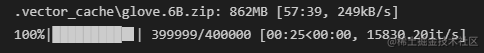## 2.3 创建迭代器+选取GPU

python BATCH_SIZE = 64

# 根据当前环境选择是否调用GPU进行训练

device = torch.device('cuda' if torch.cuda.is_available() else 'cpu')

# 创建数据迭代器

train_iterator, valid_iterator, test_iterator = data.BucketIterator.splits( (train_data, valid_data, test_data), batch_size = BATCH_SIZE, sort_within_batch = True, device = device) 

## 2.4 构建模型

### LSTM

LSTM是标准RNN的一种变体，它增加了一种携带信息跨越多个时间步的方法，一定程度上克服了标准的RNN存在梯度消失的问题。 具体来说，LSTM加入记忆单元$c_t$，其可看作是LSTM的“内存”，存储了时刻$t$时LSTM的记忆，可以认为其中保存了从过去到时刻$t$的所有必要信息，同时使用多个门控制信息流入和流出内存，具体地看此处。 因此我们可以将LSTM看作是$x_t$、$h_t$和$c_t$的函数，而不仅仅是$x_t$和$h_t$。

$$(h_t, c_t) = \text{LSTM}(x_t, h_t, c_t)$$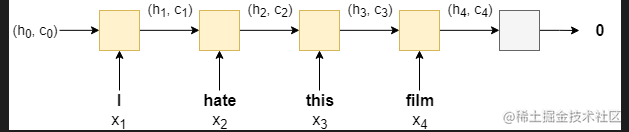### 双向RNN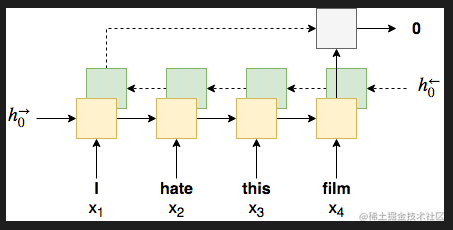### 多层RNN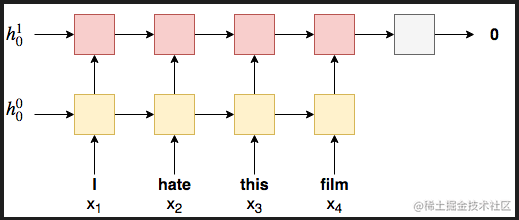### Implementation Details

2.因为实验中使用的双向LSTM的包含了前向传播和后向传播过程，所以最后的隐藏状态向量包含了前向和后向的隐藏状态，所以在下一层nn.Linear层中的输入的形状就是隐藏层维度形状的两倍。

3.在将embeddings(词向量)输入RNN前，我们需要借助nn.utils.rnn.packed_padded_sequence将它们‘打包’，以此来保证RNN只会处理不是pad的token。我们得到的输出包括packed_output (a packed sequence)以及hidden satecell state。如果没有进行‘打包’操作，那么输出的hidden statecell state大概率是来自句子的pad token。如果使用packed padded sentences，输出的就会是最后一个非padded元素的hidden statecell state

4.之后我们借助nn.utils.rnn.pad_packed_sequence 将输出的句子‘解压’转换成一个tensor张量。需要注意的是来自padding tokens的输出是零张量，通常情况下，我们只有在后续的模型中使用输出时才需要‘解压’。虽然在本案例中下不需要，这里只是为展示其步骤。

5.final hidden sate：也就是hidden，其形状是[num layers * num directions, batch size, hid dim]。因为我们只要最后的前向和后向传播的hidden states,我们只要最后2个hidden layers就行hidden[-2,:,:] 和hidden[-1,:,:]，然后将他们合并在一起,再传入线性层linear layer。

##### 这里不知道怎么解释会比较好，还需调整。

python import torch.nn as nn

class RNN(nn.Module): def init(self, vocab_size, embedding_dim, hidden_dim, output_dim, n_layers, bidirectional, dropout, pad_idx):

    super().__init__()
# embedding嵌入层（词向量）

# RNN变体——双向LSTM
self.rnn = nn.LSTM(embedding_dim,  # input_size
hidden_dim,  #output_size
num_layers=n_layers,  # 层数
bidirectional=bidirectional, #是否双向
dropout=dropout) #随机去除神经元
# 线性连接层
self.fc = nn.Linear(hidden_dim * 2, output_dim) # 因为前向传播+后向传播有两个hidden sate,且合并在一起,所以乘以2

# 随机去除神经元
self.dropout = nn.Dropout(dropout)

def forward(self, text, text_lengths):

#text 的形状 [sent len, batch size]

embedded = self.dropout(self.embedding(text))

#embedded 的形状 [sent len, batch size, emb dim]

# pack sequence
# lengths need to be on CPU!

packed_output, (hidden, cell) = self.rnn(packed_embedded)

#unpack sequence

#output的形状[sent len, batch size, hid dim * num directions]

#hidden 的形状 [num layers * num directions, batch size, hid dim]
#cell 的形状 [num layers * num directions, batch size, hid dim]

#concat the final forward (hidden[-2,:,:]) and backward (hidden[-1,:,:]) hidden layers
#and apply dropout

hidden = self.dropout(torch.cat((hidden[-2,:,:], hidden[-1,:,:]), dim = 1))

#hidden 的形状 [batch size, hid dim * num directions]

return self.fc(hidden)




## 2.5 实例化模型+传入参数

python INPUT_DIM = len(TEXT.vocab) # 250002: 之前设置的只取25000个最频繁的词,加上pad_token和unknown token EMBEDDING_DIM = 100 HIDDEN_DIM = 256 OUTPUT_DIM = 1 N_LAYERS = 2 BIDIRECTIONAL = True DROPOUT = 0.5 PAD_IDX = TEXT.vocab.stoi[TEXT.pad_token] #指定参数,定义pad_token的index索引值,让模型不管pad token

model = RNN(INPUT_DIM, EMBEDDING_DIM, HIDDEN_DIM, OUTPUT_DIM, N_LAYERS, BIDIRECTIONAL, DROPOUT, PAD_IDX) 

python def count_parameters(model): return sum(p.numel() for p in model.parameters() if p.requires_grad)

print(f'The model has {count_parameters(model):,} trainable parameters')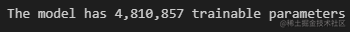python pretrained_embeddings = TEXT.vocab.vectors

# 检查词向量形状 [vocab size, embedding dim]

print(pretrained_embeddings.shape)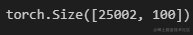python

# 用预训练的embedding词向量替换原始模型初始化的权重参数

model.embedding.weight.data.copy_(pretrained_embeddings)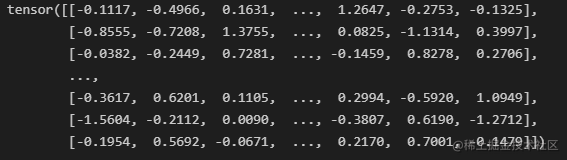python

UNK_IDX = TEXT.vocab.stoi[TEXT.unk_token]

model.embedding.weight.data[UNK_IDX] = torch.zeros(EMBEDDING_DIM) model.embedding.weight.data[PAD_IDX] = torch.zeros(EMBEDDING_DIM)

print(model.embedding.weight.data)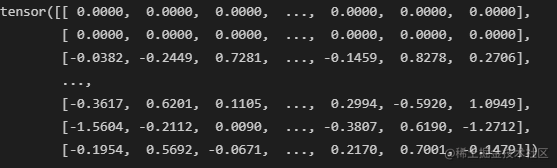## 2.6 训练模型

### 2.6.1 设置优化器

python import torch.optim as optim

optimizer = optim.Adam(model.parameters()) 

### 2.6.2 设置损失函数和GPU

python criterion = nn.BCEWithLogitsLoss() # 损失函数. criterion 在本task中时损失函数的意思

model = model.to(device) criterion = criterion.to(device) 

### 2.6.3 计算精确度

python def binary_accuracy(preds, y): """ Returns accuracy per batch, i.e. if you get 8/10 right, this returns 0.8, NOT 8 """

#round predictions to the closest integer
rounded_preds = torch.round(torch.sigmoid(preds))
correct = (rounded_preds == y).float() #convert into float for division
acc = correct.sum() / len(correct)
return acc




### 2.6.4 定义一个训练函数，用来训练模型

python def train(model, iterator, optimizer, criterion):

epoch_loss = 0
epoch_acc = 0

model.train()

for batch in iterator:

text, text_lengths = batch.text # batch.text返回的是一个元组(数字化的张量,每个句子的长度)

predictions = model(text, text_lengths).squeeze(1)

loss = criterion(predictions, batch.label)

acc = binary_accuracy(predictions, batch.label)

loss.backward()

optimizer.step()

epoch_loss += loss.item()
epoch_acc += acc.item()

return epoch_loss / len(iterator), epoch_acc / len(iterator)




### 2.6.5 定义一个测试函数

python def evaluate(model, iterator, criterion):

epoch_loss = 0
epoch_acc = 0

model.eval()

for batch in iterator:

text, text_lengths = batch.text  #batch.text返回的是一个元组(数字化的张量,每个句子的长度)

predictions = model(text, text_lengths).squeeze(1)

loss = criterion(predictions, batch.label)

acc = binary_accuracy(predictions, batch.label)

epoch_loss += loss.item()
epoch_acc += acc.item()

return epoch_loss / len(iterator), epoch_acc / len(iterator)




python import time

def epoch_time(start_time, end_time): elapsed_time = end_time - start_time elapsed_mins = int(elapsed_time / 60) elapsed_secs = int(elapsed_time - (elapsed_mins * 60)) return elapsed_mins, elapsed_secs 

### 2.6.6 正式训练模型

python N_EPOCHS = 5

best_valid_loss = float('inf')

for epoch in range(N_EPOCHS):

start_time = time.time()

train_loss, train_acc = train(model, train_iterator, optimizer, criterion)
valid_loss, valid_acc = evaluate(model, valid_iterator, criterion)

end_time = time.time()

epoch_mins, epoch_secs = epoch_time(start_time, end_time)
# 保留最好的训练结果的那个模型参数，之后加载这个进行预测
if valid_loss < best_valid_loss:
best_valid_loss = valid_loss
torch.save(model.state_dict(), 'tut2-model.pt')

print(f'Epoch: {epoch+1:02} | Epoch Time: {epoch_mins}m {epoch_secs}s')
print(f'\tTrain Loss: {train_loss:.3f} | Train Acc: {train_acc*100:.2f}%')
print(f'\t Val. Loss: {valid_loss:.3f} |  Val. Acc: {valid_acc*100:.2f}%')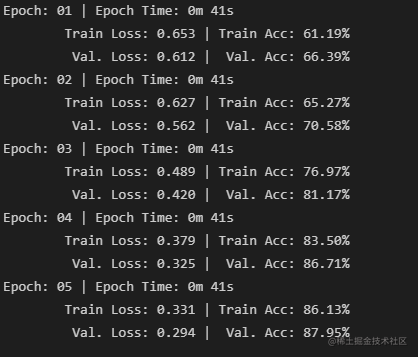### 2.6.7 最终测试结果

python model.load_state_dict(torch.load('tut2-model.pt'))

test_loss, test_acc = evaluate(model, test_iterator, criterion)

print(f'Test Loss: {test_loss:.3f} | Test Acc: {test_acc*100:.2f}%') 

Test Loss: 0.334 | Test Acc: 85.28%


## 2.7 模型验证

“predict_sentiment”函数的作用如下：

• 将模型切换为evaluate模式
• 对句子进行分词操作
• 将分词后的每个词，对应着词汇表，转换成对应的index索引，
• 获取句子的长度
• 将indexes，从list转化成tensor
• 通过unsqueezing 添加一个batch维度
• 将length转化成张量tensor
• 用sigmoid函数将预测值压缩到0-1之间
• 用item（）方法，将只有一个值的张量tensor转化成整数

python import spacy nlp = spacy.load('en_core_web_sm')

def predict_sentiment(model, sentence): model.eval() tokenized = [tok.text for tok in nlp.tokenizer(sentence)] indexed = [TEXT.vocab.stoi[t] for t in tokenized] length = [len(indexed)] tensor = torch.LongTensor(indexed).to(device) tensor = tensor.unsqueeze(1) length_tensor = torch.LongTensor(length) prediction = torch.sigmoid(model(tensor, length_tensor)) return prediction.item() 

python predict_sentiment(model, "This film is terrible")

0.05380420759320259


python predict_sentiment(model, "This film is great")

0.94941645860672


## 小结

#### 参考资料

https://blog.csdn.net/weixin_42167712/article/details/112196925

「其他文章」